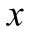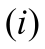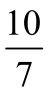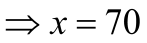A student scored 49 on a test. He received a 70 percent on his report card. What is the test’s highest possible score?

# A student scored 49 on a test. He received a 70 percent on his report card. What is the test's highest possible score?

1. A
60
2. B
70
3. C
80
4. D
None of these

Fill Out the Form for Expert Academic Guidance!l

+91

Live ClassesBooksTest SeriesSelf Learning

Verify OTP Code (required)

### Solution:

Concept: We can use the straightforward concept of % to answer the question. We must initially assume that the test's overall grade is x. To get the grade that the student received in terms of x, we must convert the given percentage into a fraction and multiply it by x. For example, if we have total data of n items and we take a sample of m items from total data of n items, sample over total data equals the ratio of amount of sample from total data, and when ratio is multiplied by 100, the expression we get is percentage of sample m in total data n. In order to solve the equation, we finally equate the expression to 49 to get the value of x.
Consider that the test's overall grade is.
As far as we are aware, a percentage is a figure or ratio that denotes a portion of one hundred. Twenty percent, for instance,Similarly, 70% is represented as.
His score was 70 on the report card, which signifies that he scored 70 of, in accordance with the question. His mark is thus determined by.
However, assuming that his score was 49, we obtainNow we may find the answer by multiplying both sides of equationby.Here, we received a score of 70 overall.
Hence, the correct option is 2) 70

## Related content

 Area of Square Area of Isosceles Triangle Pythagoras Theorem Triangle Formula Perimeter of Triangle Formula Area Formulae Volume of Cone Formula Matrices and Determinants_mathematics Critical Points Solved Examples Type of relations_mathematics+91

Live ClassesBooksTest SeriesSelf Learning

Verify OTP Code (required)